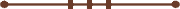####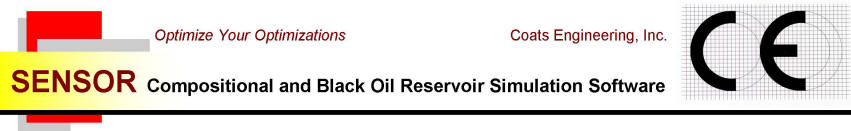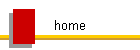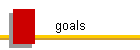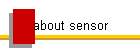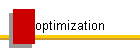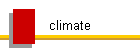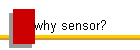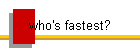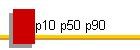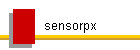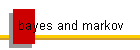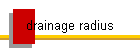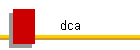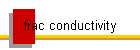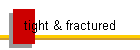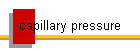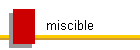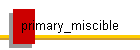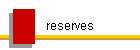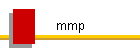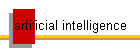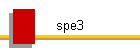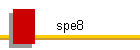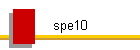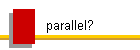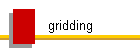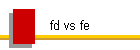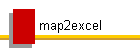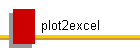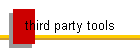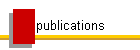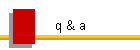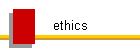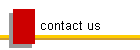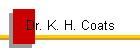#### A Unidirectional Flow Problem

The case presented here for single phase incompressible flow tests the ability of the numerical model to obtain the correct unidirectional flow rate for an anisotropic 2D rectangular reservoir with constant pressure boundary conditions.

The horizontal reservoir is 10000 ft long (x-direction) and 2000 ft wide (y-direction).  The grid is described with Nx=20, Ny=10, Nz=1, dx=500 ft, dy=200 ft, dz=1000 ft, all block depths (to top) at 8000 ft, kx=10 md, ky=2 md, kz=10 md.  Initial pressure is 5000 psia.  The left (i=1) boundary is constrained to 5100 psia, and the right boundary (i=nx) is constrained to 4900 psia.  PVT and other data are based on SPE1.  The correct steady state rate given by Darcy's law and reproduced by Sensor in the output file given below, independent of the entered permeability in the direction perpendicular to flow (ky), is

(kA/L) * dp = (10(1000)(2000)/(19*500)) * 200 *.00633/5.6146 = 474.7 stb/d

kx   Lz       Ly          Lx          dp     conversion

Sensor can obtain the same result using as little as 2 total grid cells.  4 cells are required to demonstrate independence of results on entered ky.

Sensor data:   uni.dat

Sensor output: uni.out

This problem is a test for k-orthogonality and will demonstrate:

• An exact Finite Difference solution using a Cartesian grid, independent of ky.

• The requirement for use of a multipoint flux approximation, and its accuracy, when solved with unstructured grids using, for example, hexagonal prisms, or with any grid that is not orthogonal in the direction of flow, for example, corner point grids differing from Cartesian geometry.

• Smooth boundary approximation issues with unstructured grids not using rectangular cells or mesh.

• The requirement for a Cartesian grid or equivalent finite element rectangular mesh in order to prevent grid orientation effects that otherwise cause transverse dispersion of a tracer injected at a point on the left boundary.  This illustrates that all fixed grids can suffer from grid orientation effects, even if they are k-orthogonal.

© 2000 - 2022 Coats Engineering, Inc.